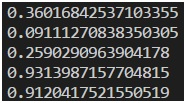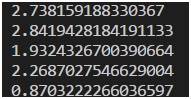# Playing with Number in JavaScript

We will create a rolling dice program while discussing all related term used in it.
Submitted by Himanshu Bhatt, on September 04, 2018

float

Before getting started with creating a rolling die program, let's recall what we know about floats? In simplest words, Floats are the decimal numbers. Let’s see an example:

```
console.log(22/7)
```

Output

`3.142857142857143`

toFixed method

Now sometimes we don’t need numbers with many decimal places (or precision) so we try following:

```    result = 22/7
console.log(result.toFixed(2))
```

Now we created a result and used a predefined method toFixed which takes a digit (optional) and returns the round off of the number to that digit passed as an argument.

Syntax:

```    number.toFixed(digit);
```

Where a digit can be from 0 to 20 (inclusive).

Example:

```result = 22/7
console.log(result.toFixed(2)) //line 1
console.log(result.toFixed()) //line 2
console.log(result.toFixed(5)); //line 3
```

Here we have a code snippet we passed toFixed method with 3 different arguments, as we can see that we have 2, "no argument' equivalent to 0 and 5 and see the respective outputs below.

```    3.14
3
3.14286
```

Math

For making a rolling dice we will be needing some functions from math.

1. Floor method

Floor method will return downwards to largest number. Let’s see some examples:

```console.log(Math.floor(34.323243))  //line 1
console.log(Math.floor(99.9999999999)) //line 2
```
```    The output of Line 1: 34
The output of Line 2: 99
```

2. Ceil Method

Ceil method will return the upwards to the smallest number. Let’s see some examples:

```console.log(Math.ceil(34.323243))	//line 1
console.log(Math.ceil(99.9999999999))	//line 2
```
```    The output of Line 1: 35
The output of Line 2: 100
```

3. Random method

This method is pretty handy for our purpose because it returns a random number between 0 and 1. But, how can we need to produce a number between 1 to 6 let’s see an example first:

Example:

```for(i=0;i<5;i++){
myRandomNumber =Math.random()
console.log(myRandomNumber);
}
```

Now we use a for loop for getting 5 random numbers.

Now the following output is after we ran the above code snippet:As we can see that all the 5 randomly generated values are between 0 and 1. So now what we will do is:

```for(i=0;i<5;i++){
myRandomNumber =Math.random()*3
console.log(myRandomNumber);
}
```

Notice that we multiply the older expression with a number (in above example it’s 3).

Output:So our output has changed from values between 0 to 1 to values 0 to 3 (of course, upper limit excluded).

Now we make the final version of our Dice Rolling Program with JavaScript, and here is the Code:

Rolling Dice program:

```upper = 6
lower = 0
for(i=0;i<10;i++){
myRandomNumber =Math.ceil(Math.random()*(upper-lower)+lower)	//line 1
console.log(myRandomNumber);
}
```

Explanation:

In Line 1, we used Math.random() to produce a number between 0 and 1 then we used lower and upper limit variables to make our code more generalized. So we multiplied the Math.random() with (upper – lower), so we get the number in that range [but mathematically, it’s 6 in our case (6-0)] so it will produce numbers between 0 and 5.99x (well it’ll work for our case but in sake of generalizing thing and we want random numbers between lower and upper so we will add lower to it, but still the number has decimal so we used ceil method [and not floor because it will result in values between 0 to 5] to round it off.

Top MCQs

Languages: » C » C++ » C++ STL » Java » Data Structure » C#.Net » Android » Kotlin » SQL
Web Technologies: » PHP » Python » JavaScript » CSS » Ajax » Node.js » Web programming/HTML
Solved programs: » C » C++ » DS » Java » C#
Aptitude que. & ans.: » C » C++ » Java » DBMS
Interview que. & ans.: » C » Embedded C » Java » SEO » HR
CS Subjects: » CS Basics » O.S. » Networks » DBMS » Embedded Systems » Cloud Computing
» Machine learning » CS Organizations » Linux » DOS
More: » Articles » Puzzles » News/Updates